Open in App
Not now

# How to Create Formula Cell in Excel Sheet using Java and Apache POI?

• Last Updated : 19 May, 2022

In the previous article, we have seen how to read data from the formula cell, here we are going to create the formula cell using Apache POI.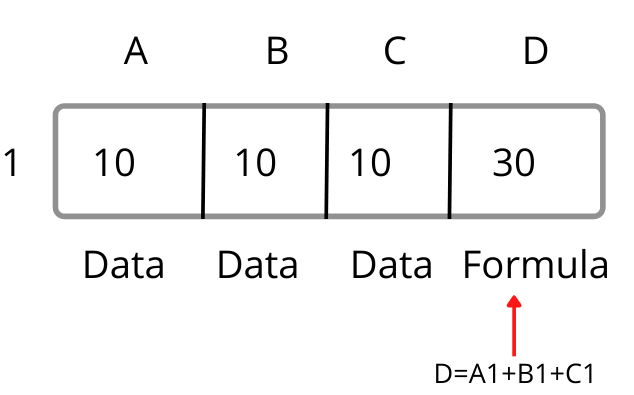In this article, we are creating an Excel file with three columns consisting of values and the last column is the formula cell which is calculated from the other cells by defining a certain formula, here we are creating the formula cell by adding all other data in the cells.

Pre-requisites:

To work will these, we needed the following software in your systems.

• Create the Maven project.

For creating the maven project refer to this article on how to create a selenium maven project with eclipse.

• Add the Apache POI dependency into the POM.xml file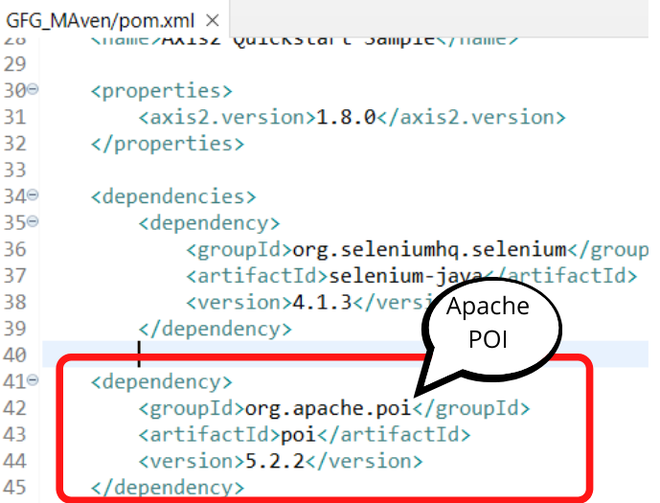## Java

 `package` `GFG_Maven.GFG_MAven; ` ` `  `import` `org.testng.annotations.Test; ` ` `  `import` `java.io.FileInputStream; ` `import` `java.io.FileOutputStream; ` `import` `java.io.IOException; ` ` `  `import` `org.apache.poi.xssf.usermodel.XSSFCell; ` `import` `org.apache.poi.xssf.usermodel.XSSFRow; ` `import` `org.apache.poi.xssf.usermodel.XSSFSheet; ` `import` `org.apache.poi.xssf.usermodel.XSSFWorkbook; ` ` `  `public` `class` `Geeks { ` `    ``@Test` `    ``public` `void` `geekforgeeks() ``throws` `IOException{ ` ` `  `        ``XSSFWorkbook workbook=``new` `XSSFWorkbook(); ` `        ``XSSFSheet sheet=workbook.createSheet(``"Numbers"``); ` `         `  `        ``XSSFRow row=sheet.createRow(``0``); ` `         `  `        ``row.createCell(``0``).setCellValue(``10``); ` `        ``row.createCell(``1``).setCellValue(``10``); ` `        ``row.createCell(``2``).setCellValue(``10``); ` `         `  `        ``row.createCell(``3``).setCellFormula(``"A1+B1+C1"``); ` `         `  `        ``FileOutputStream file = ``new` `FileOutputStream(``"C:\\Users\\ADMIN\\Desktop\\calc.xlsx"``); ` `        ``workbook.write(file); ` `        ``file.close(); ` `        ``System.out.println(``"calc.xlsx file is created with Formula cell"``); ` `        `  `    ``} ` `}`

### Code Explanation:

After creating the workbook, we have created the sheet in the workbook as “Numbers”.

XSSFSheet sheet=workbook.createSheet(“Numbers”)

Then we have created the first row in that sheet by the createRow() method.

XSSFRow row=sheet.createRow(0);

After that, we have created the cells in the first row by row.createCell(cell number).setCellValue(value);

row.createCell(0).setCellValue(10);

Now, in the fourth cell, we are using the formula to calculate the cell value by using the setCellFormula(formula).

row.createCell(3).setCellFormula(“A1+B1+C1”);

In the end, we are going to write the values into the Excel sheet by creating a file output stream into the defined location

FileOutputStream file = new FileOutputStream(“specify the location for creating the file”);

Writing the cell values into the file by workbook.write(file),

### Output:

After we run the code, we get the output and the Excel files are created.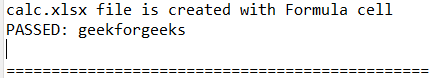Now we can notice that the Excel file is created in the defined location. I have given desktop location, so the file is created on my desktop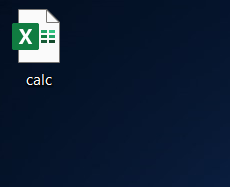If you open the Excel file the cell values are created in the sheet with the formula cell.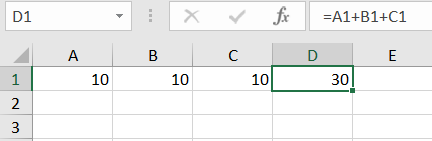My Personal Notes arrow_drop_up
Related Articles